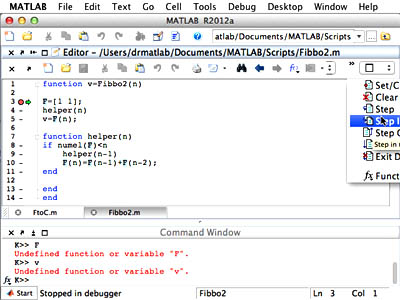18.S997 | Fall 2011 | Undergraduate
Introduction To MATLAB Programming

## Debugging with MATLABDebugging code in MATLAB helps ensure that code produces the desire output.

At some point you will realize that MATLAB® is not doing what you want it to do. This is normally due to a mistake which is either that you don’t understand how MATLAB works or that you have a typo that MATLAB understands in a way different form what you want it to be. In any case, it is a bug (in your code, as opposed to a bug in MATLAB, which is less likely the culprit), and can normally be traced to one particular line of code that has a typo in it. MATLAB has a “debugger” that helps you locate and correct that line of code.

The debugger allows you to set breakpoints (that tell MATLAB to pause in a kind of “suspended animation” when it reaches that line. You can then tell MATLAB to execute the code one step at a time. When MATLAB is stopped in debugging mode you can do several things:

• Examine/change the value of variables
• Look at the “stack” of functions
• Evaluate arbitrary expressions (possibly changing the values of variables when you do)
• Add, remove, or modify breakpoints

The first thing you may want to do is “set” a breakpoint. Do this at the line that is just before where you suspect something is wrong. You set breakpoints by clicking on the ‘-’ (minus) sign next to the line number in the MATLAB file editor. If all is well a red circle will appear indicating that MATLAB will stop just before the next time it evaluates that line. Now when you run your program you will see a green arrow on that line. The arrow indicates MATLAB’s current location in the execution path. You will also see that your command prompt is now `K>>`. This is to remind you that you are currently debugging so all the variables you see are “local.” You can now click on the various debugging menu items (Step, Step into, Step out, etc.) and see what they do. While at the debugging prompt, you can change the variables but not create new ones. You can also view the values of variables by typing their names on the prompt. In fact, you can type any expression and MATLAB will evaluate it as if the expression were given in the file that is being debugged. So you can try a few things out until you find the right one, and only then copy-paste that expression into the file.

For debugging there’s really no substitute to trying it out. Once you have some code that is behaving strangely, try debugging it using the debugger.

The video below demonstrates, step-by-step, how to work with MATLAB in relation to the topics covered in this unit.